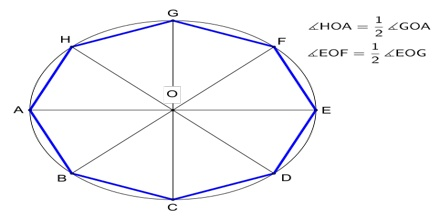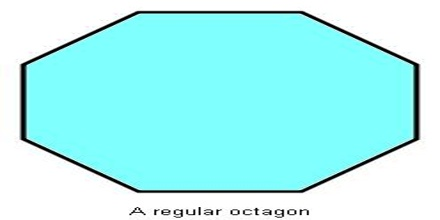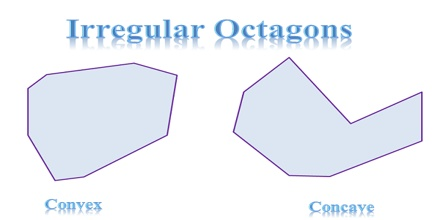Mathematic

# Octagon Polygon: Overview and TypesOctagon is a polygon which has 8 sides is known as an octagon. It is a two-dimensional closed shape. Octagons must have straight sides that connect; they cannot be curved or disconnected. The word “octagon” is made up of octa + gonia which are Greek words. Octa means eight and gonia is referred to angle. An octagon has 8 sides and 8 internal angles.Properties of an Octagon

There are following important properties of an octagon:

• It has eight sides.
• It has eight angles.
• Sum of interior angles of octagon is (8 – 2) 1800, i.e. 6 x 1800 which is equal to 10800.
• Sum of exterior angles of an octagon is 3600.

Octagon Types

Regular Octagon

An octagon which has eight equal sides as well as eight equal interior angles, is called a regular octagon.Irregular octagons and concave octagon

Irregular octagons have sides that are not congruent, which means that they aren’t all the same measurement. They can also have points that are facing inward and outward. An octagon that has points that are facing inward is a concave octagon.Properties of a Regular Octagon

The properties of regular octagon are:

• It has eight equal sides.
• It has all internal angles equal in measure.
• Each interior angle measures 10800 / 8 or 1350.
• Each exterior angles measures 3600 / 8 or 450.